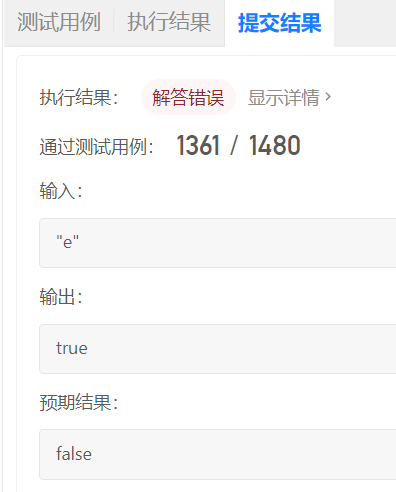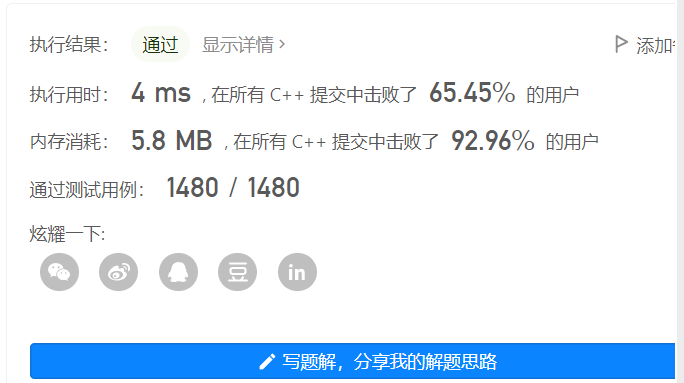# 前言# 一、憨憨初解

## 2、代码

class Solution {
public:
bool isNumber(string s) {
if (s.empty() || s.size() == 0) return false;
int cur=0;
while(s[cur] == ' ')  cur++;//去调首部空格
//处理一个整数或者小数
if(s[cur] == '+' || s[cur] == '-') cur++;
while(s[cur] >= '0' && s[cur] <= '9')    cur++;
if(s[cur] == '.') cur++;
while(s[cur] >= '0' && s[cur] <= '9')    cur++;

//处理e和E以及一个整数
if(s[cur]=='e' || s[cur]=='E') cur++;
if(s[cur] == '+' || s[cur] == '-') cur++;
while(s[cur] >= '0' && s[cur] <= '9')    cur++;

while(s[cur] == ' ')  cur++;//处理尾部空格
return cur==s.size();
}
};


## 3、战绩# 二、看懂再解

## 1、思路

1、小数点出现的时候，前面不能出现过小数点或者是出现过e；

• 解释：'.'只能在底数上，不能在指数上，且只能出现一次，'.'两边任一边有数字均算一个完整的数字，但单独一个'.'不行。

2、e或者E出现的时候，前面不能出现过eE，并且必须出现过一个数字；

• 解释：e、E用来划分底数与指数，只能出现一次，前面为科学计数法的底数，后面为指数；

3、+-符号，只能出现在第一位或者紧接e后面；

• 解释：'+'、'-'只能作为正负号出现在底数和指数的前面，不能出现在两个数字中间；

## 2、代码

class Solution {
public:
static void trim(string &s){
if( !s.empty() ){
s.erase(0,s.find_first_not_of(" "));
s.erase(s.find_last_not_of(" ") + 1);
}
}
bool isNumber(string s) {
if (s.empty() || s.size() == 0) return false;
trim(s);
bool is_num = false;//判断数字是否出现过
bool is_dot = false;//判断.是否出现过
bool is_eorE = false;//判断e或者E是否出现过
int i = 0,size = s.size();
for (; i < size; i++) {
//判定为数字，则标记is_num
if (s[i] >= '0' && s[i] <= '9') {
is_num = true;
//判定为.  需要前面没出现过.并且没出现过e
} else if (s[i] == '.' && !is_dot && !is_eorE) {
is_dot = true;
//判定为e或者E，需要前面没出现过e，并且出现过数字
} else if ((s[i]=='e' || s[i]=='E') && !is_eorE && is_num) {
is_eorE = true;
is_num = false;//为了避免123e这种请求，出现e之后就标志为false
//判定为+-符号，只能出现在第一位或者紧接e后面
} else if (s[i] == '-' || s[i] == '+'){
if(i!=0 && s[i-1] != 'e' && s[i-1] != 'E'){
return false;
}
} else {
return false;
}
}
return is_num;
}
};


class Solution {
public boolean isNumber(String s) {
//有限状态机
// 2.小数点 3.E/e 4. 数字字符 5. -+
if(s == null || s.length() <= 0){
return false;
}
char[] res = s.trim().toCharArray();
if(res.length <= 0) return false;

int n = res.length;

boolean is_dot = false;
boolean is_e_or_E = false;
boolean is_num = false;
for(int i = 0; i < n; i++){
if(res[i] >= '0' && res[i] <= '9'){
is_num = true;
} else if(res[i] == '.'){
//-+ 8.  8.8  .8
// 前面：不能有重复的小数点，也不能出现 e/E
if(is_dot || is_e_or_E){
return false;
}
is_dot = true;
} else if(res[i] == 'e' || res[i] == 'E'){
// 前面必须要有一个数字 || 前面不能出现重复的 e/E
if(is_e_or_E || !is_num){
return false;
}
is_e_or_E = true;
is_num =false;//11E+ 11E
} else if(res[i] == '-' || res[i] == '+'){
if(i!=0 && res[i-1] != 'e' && res[i-1] != 'E'){
return false;
}
} else {
return false;
}
}

return is_num;
}
}



## 3、C++版战绩# 总结

posted @ 2022-11-04 18:15  BingeBlog  阅读(270)  评论(0编辑  收藏  举报